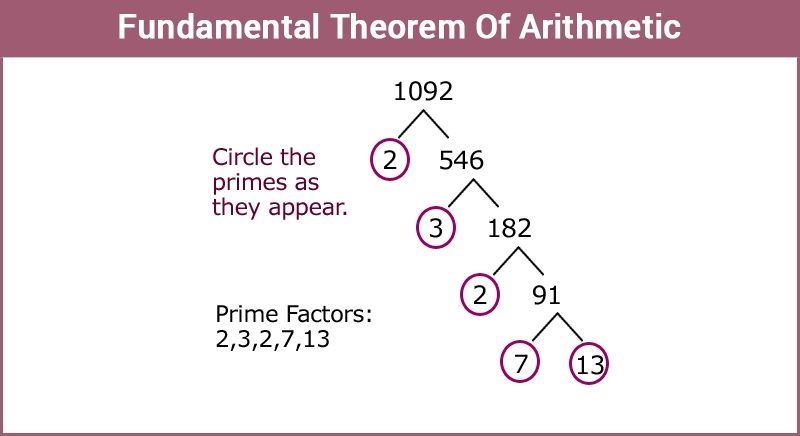# Fundamental Theorem Of Arithmetic

Fundamental Theorem of Arithmetic states that every integer greater than 1 is either a prime number or can be expressed in the form of primes. In other words, all the natural numbers can be expressed in the form of the product of its prime factors. To recall, prime factors are the numbers which are divisible by 1 and itself only. For example, the number 35 can be written in the form of its prime factors as:

35 = 7 × 5

Here, 7 and 5 are the prime factors of 35

Similarly, another number 114560 can be represented as the product of its prime factors by using prime factorization method,

114560 = 27 × 5 × 179

So, we have factorized 114560 as the product of the power of its primes.

Therefore, every natural number can be expressed in the form of the product of the power of its primes. This statement is known as the Fundamental Theorem of Arithmetic, unique factorization theorem or the unique-prime-factorization theorem.## Proof for Fundamental Theorem of Arithmetic

In number theory, a composite number is expressed in the form of the product of primes and this factorization is unique apart from the order in which the prime factor occurs.

From this theorem we can also see that not only a composite number can be factorized as the product of their primes but also for each composite number the factorization is unique, not taking into consideration order of occurrence of the prime factors.

In simple words, there exists only a single way to represent a natural number by the product of prime factors. This fact can also be stated as:

The prime factorization of any natural number is said to be unique for except the order of their factors.

In general, a composite number “a” can be expressed as,

a = p1 p2 p3 ………… pn, where p1, p2, p3 ………… pn are the prime factors of a written in ascending order i.e. p1≤p2≤p3 ………… ≤pn.

Writing the primes in ascending order makes the factorization unique in nature.

### Fundamental Theorem of Arithmetic Examples

Example Question: In a formula racing competition the time taken by two racing cars A and B to complete 1 round of the track is 30 minutes and 45 minutes respectively. After how much time will the cars meet again at the starting point?

Solution:

As the time taken by car B is more compared to that of A to complete one round therefore it can be assumed that A will reach early and both the cars will meet again when A has already reached the starting point. This time can be calculated by finding the L.C.M of the time taken by each.

30 = 2 × 3 × 5

45 = 3 × 3 × 5

The L.C.M is 90.

Thus, both cars will meet at the starting point after 90 minutes.

### Related Video Lesson: